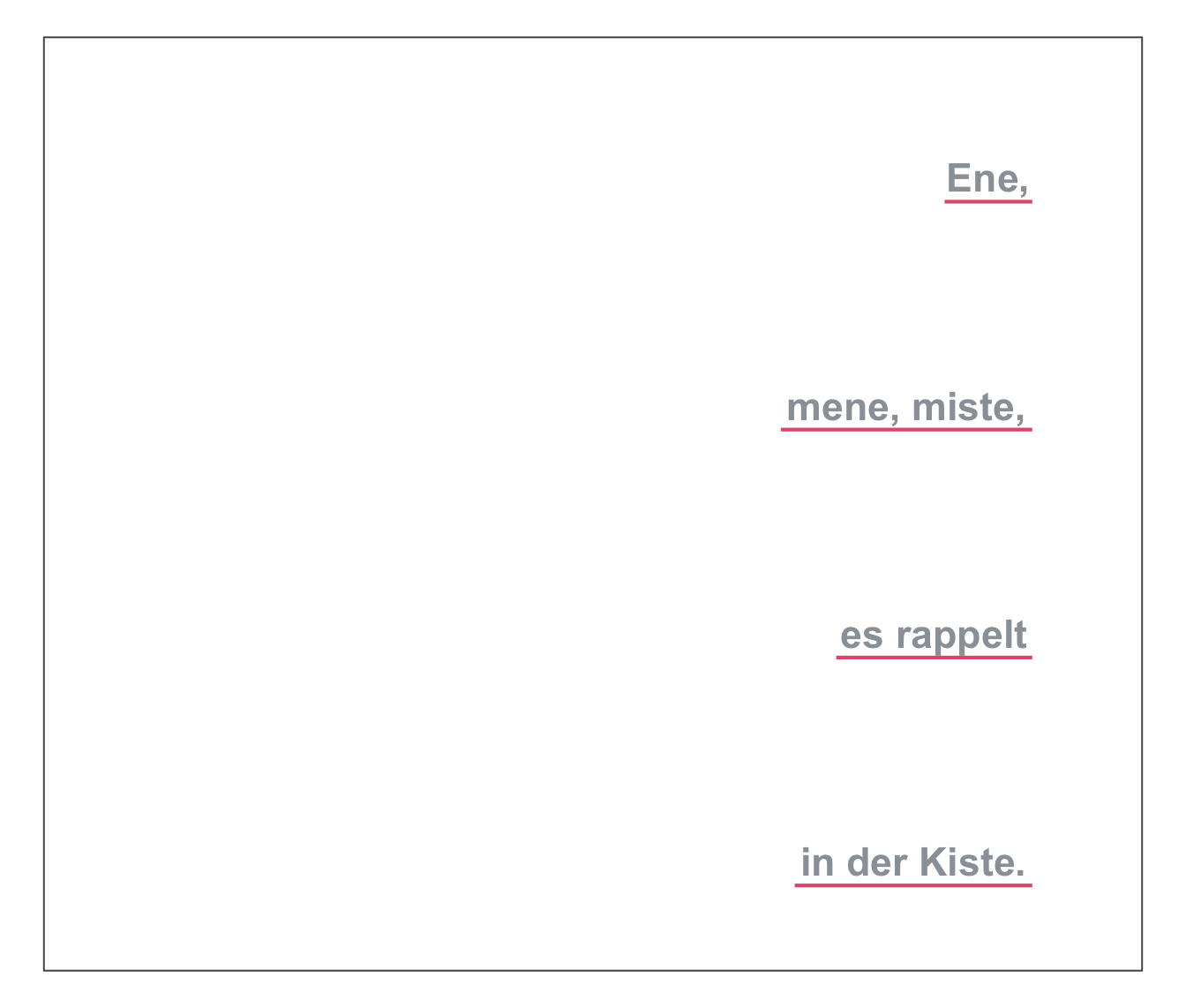uline plots 1 or more text strings (provided as a character vector labels) to an (existing or new) plot and places a colored line underneath each label (to underline it).

uline(
labels,
x = 0,
y = 0.55,
x_layout = NA,
y_layout = "even",
col = "black",
col_bg = Seeblau,
cex = 1.5,
font = 1,
new_plot = "none"
)

## Arguments

labels A character vector specifying the text labels to be plotted. A numeric vector of x-coordinates at which the text labels in labels should be written. If the lengths of x and y differ, the shorter one is recycled. Default: x = 0. A numeric vector of y-coordinates at which the text labels in labels should be written. If the lengths of x and y differ, the shorter one is recycled. Default: y = .55. An optional numeric vector or character string to control the horizontal positions of labels. Numeric values are interpreted as increments to values of x and recycled (to enable stepwise or alternating patterns). 3 character string options are: "center" (i.e., center wrt. first label or plot center), "left" (i.e., left wrt. first label or plot center), "right" (i.e., right wrt. first label or plot center). Default: x_layout = NA (i.e., using values of x). A numeric value or character string to control the vertical positions of labels. Numeric values are interpreted as increments to values of y and recycled (to enable stepwise or alternating patterns). 2 character string options are: "even" (i.e., even distribution of labels across available y-space) and "flush" (i.e., no space between adjacent labels, i.e., y_layout = 0). Default: y_layout = "even". The color(s) of the text label(s). Default: col_lbl = "black". The color(s) of the line (under the text labels of labels). Default: col_bg = Seeblau. Numeric character expansion factor(s), multiplied by par("cex") to yield the character size(s). Default: cex = 1.5. The font type(s) to be used. Default: font = 1 (i.e., plain text). Boolean: Should a new plot be generated? Set to "blank" or "slide" to create a new plot. Default: new_plot = "none" (i.e., add to an existing plot).

## Details

The positions of the text elements in labels can be specified by providing their coordinates (as x and y arguments) or by providing an initial position and an y_layout (see below).

Text formatting parameters (like col, col_bg, cex, font) are recycled to match length(labels).

uline uses the base graphics system graphics::.

slide and xbox to create simple plots (without text).

Other text functions: mark(), post(), url_unikn()

## Examples

uline(labels = "This is a test.", new_plot = "blank")  # create a new blank plot
uline(labels = "More testing here...", y = .33, col_bg = pal_pinky[])  # add to plot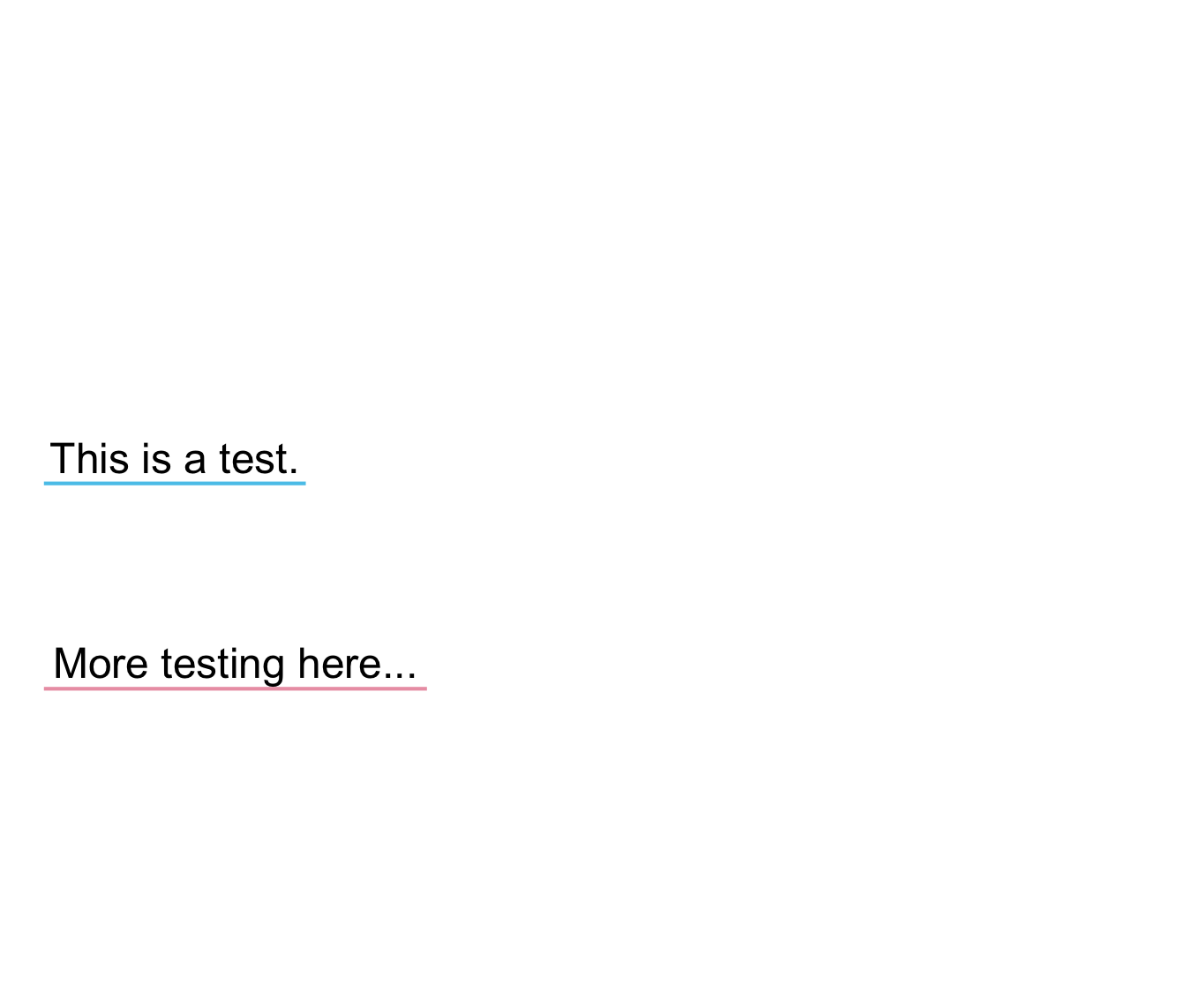# 2 basic cases:
# (a) Underline text on an existing plot:
plot(x = 0, y = 0, type = "n", xlim = c(0, 1), ylim = c(0, 1), xlab = "", ylab = "")
uline(x = 0, y = .8, labels = "Underline text (on an existing plot)")  # add to plot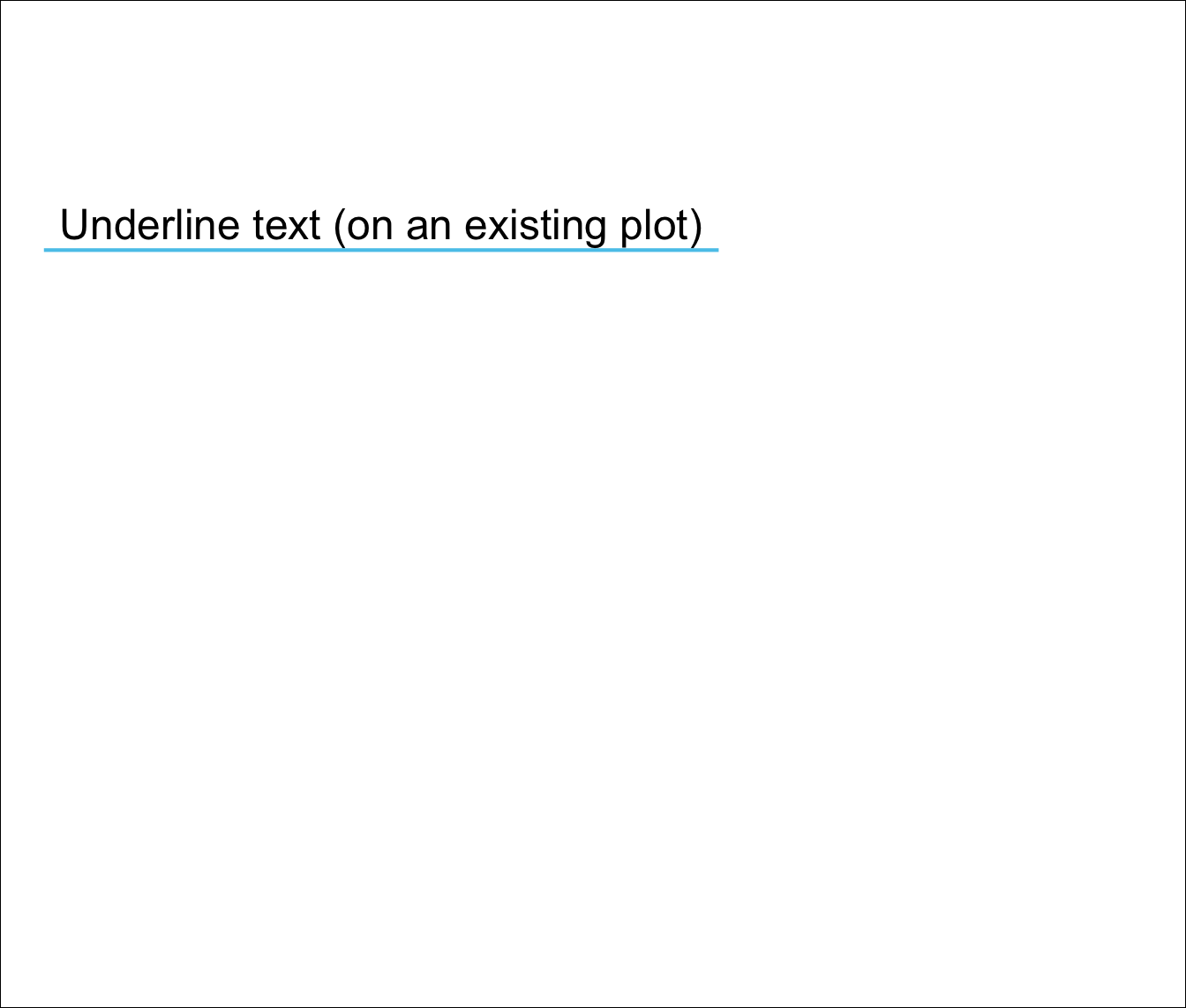# (b) Underline text on a new plot:
uline(x = .02, y = .80, labels = "Underline text (on a new plot)",
new_plot = "slide")  # create a new plot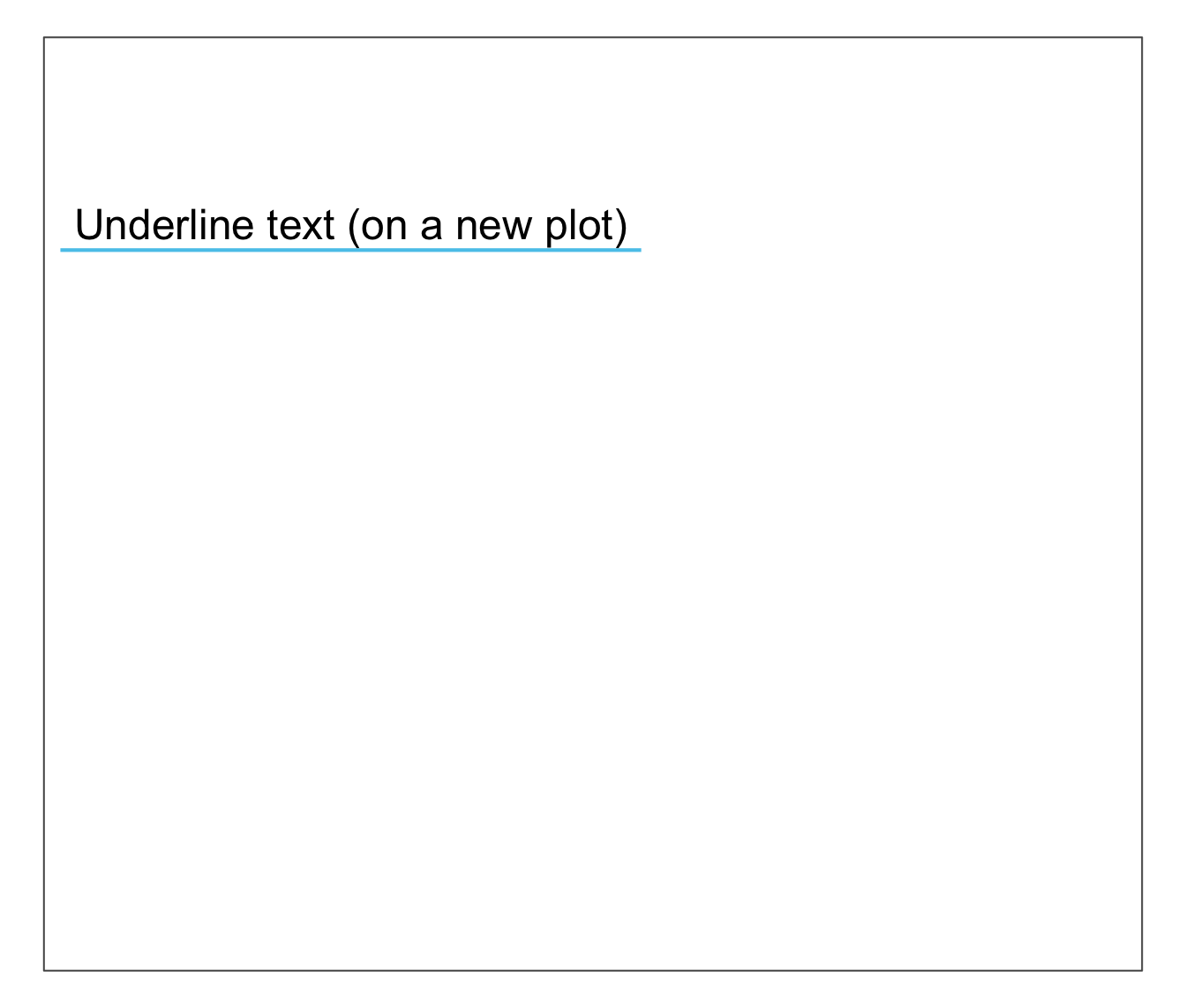# Example:
lbl_line <- c("This is neat, true, and terribly important.")
uline(labels = lbl_line, new_plot = "blank")  # create a new plot
uline(labels = "(which is why we underline it).", y = .40, cex = 1.2)  # add to plot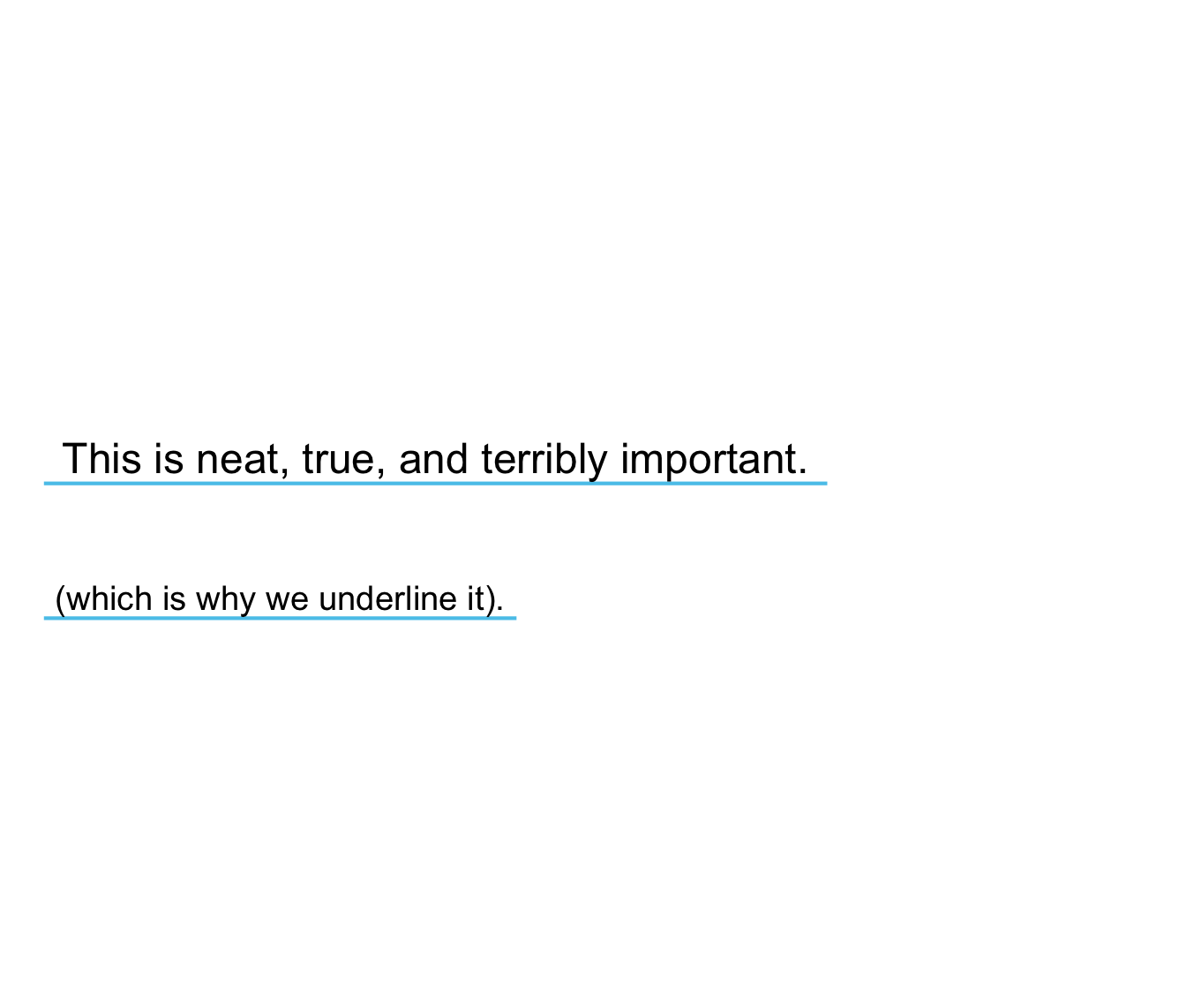# Using x_layout and y_layout:
uline(labels = c("Ene,", "mene, miste,", "es rappelt", "in der Kiste."),
cex = 1.4, font = 2, col = Grau, col_bg = Pinky,
x = 1.2, y = .85, x_layout = "right", y_layout = "even", new_plot = "slide")